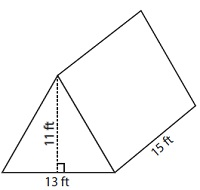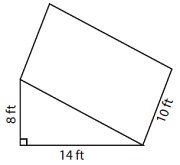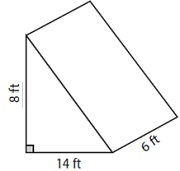# Volume of Triangular Prism

In this lesson we find the volume of a triangular prism

A triangular prism is a prism that has two congruent parallel triangles as its bases and rectangular lateral faces.Formula for the volume of a triangular prism

If A is the area of the base triangle and h is the height of the prism then volume of the prism is given by

Volume V = A × h

Where A = $\frac{1}{2}$ b h or $\sqrt{s(s-a)(s-b)(s-c)}$ or $a^2 \sqrt{3}/ 4$

b is the base of the triangle and h is the height

a, b, and c are the sides of the triangle and s =(a+b+c)/2

a is the side of an equilateral triangle

Find the volume of the following triangular prism.### Solution

Step 1:

Volume of a triangular prism = Areas of base triangle × height of prism

Step 2:

Volume of given prism V = $\frac{1}{2}$ × 14 × 8 × 10

= 560 cubic feet

Find the volume of the following triangular prism.### Solution

Step 1:

Volume of a triangular prism = Areas of base triangle × height of prism

Step 2:

Volume of given prism V = $\frac{1}{2}$ × 14 × 8 × 6

= 336 cubic ft